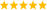# UI Interest Group Discussions

cancel
Showing results for
Did you mean:

Highlighted

## Is there a way to enter math expression from the front panel and visualize the formula?

I recently need to allow user to enter math expression from the front panel and programmatically make the formula to let them create new signal accordingly. It is the first challenge.

The other challenge (in related to UI), is there a way to visualize the equation on the front panel based on the entered math expression? (like the following bellow)Message 1 of 4
(238 Views)
Highlighted

## Re: Is there a way to enter math expression from the front panel and visualize the formula?

LabVIEW has formula parser tools (details). See also the links in this article . There is also the gpower toolkit and others such as this one. and this one.LabVIEW Champion. It all comes together inGCentralWhat does "Engineering Redefined" mean??
Message 2 of 4
(173 Views)
Highlighted

## Re: Is there a way to enter math expression from the front panel and visualize the formula?

@altenbach wrote:

LabVIEW has formula parser tools (details). See also the links in this article . There is also the gpower toolkit and others such as this one. and this one.

Thank you for the formula parser tools. It's awesome.

My concern in this post is how to visualize the math expression entered by user, to be shown as mathEquation/formula on Front Panel. (Programmatically, works in runtime)

Message 3 of 4
(127 Views)
Highlighted

## Re: Is there a way to enter math expression from the front panel and visualize the formula?

@altenbach wrote:

I found Darin's Math Node there. looks interesting.

Now I'm thinking how to make it work vise-versa.

user enter the math expression (e.g. Gpower) and programmatically display the equation (math formula) under labview run time.

Some body have any Idea?

Message 4 of 4
(63 Views)